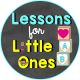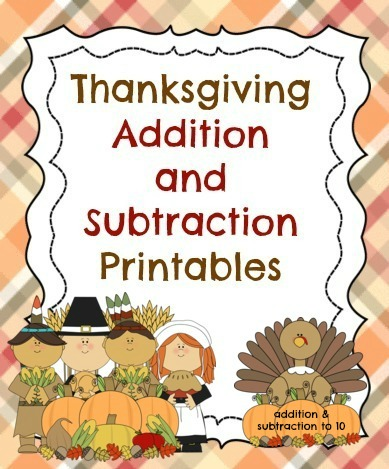# Thanksgiving Addition to 10, Subtraction to 10 Printable PagesPosted by# Thanksgiving Addition to 10, Subtraction to 10 Printable Pages

A variety of Thanksgiving themed reproducible pages for practicing addition to 5, addition to 10, subtraction to 5, and subtraction to 10. Provides multiple activities featuring cute Thanksgiving characters and fun formats that will keep students engaged and interested. A simple and easy addition to your Thanksgiving curriculum.

This packet provides addition and subtraction to 10 practice in a variety of formats and ability levels.

They look at pictures that match the problems and write the sum.

They look at pictures that match the problems and write the entire addition problem.

They solve addition problems with no pictures using Pilgrim counters and a Mayflower mat for assistance.

Cut and paste activity – they match pictures to numbers in an addition problem and write the sum.

They look at pictures that match the problems and write the sum. They look at pictures that match the problems and write the entire addition problem.

They draw pictures to match the addition problem and write the sum. They complete a number sentence that equals 10.

Subtraction to 10:
They solve subtraction problems by crossing out the appropriate number of turkeys (subtraction to 5).

They solve subtraction problems by crossing out the appropriate number of Pilgrim hats (subtraction to 10).

They solve subtraction to 10 problems with no pictures using Thanksgiving food counters and a dinner table mat for assistance.

These printable pages can be used to practice the following Common Core Standards:
CCSS.MATH.CONTENT.K.OA.A.1
Represent addition and subtraction with objects, fingers, mental images, drawings1, sounds (e.g., claps), acting out situations, verbal explanations, expressions, or equations.
CCSS.MATH.CONTENT.K.OA.A.2
Solve addition and subtraction word problems, and add and subtract within 10, e.g., by using objects or drawings to represent the problem.
CCSS.MATH.CONTENT.K.OA.A.4
For any number from 1 to 9, find the number that makes 10 when added to the given number, e.g., by using objects or drawings, and record the answer with a drawing or equation.
CCSS.MATH.CONTENT.K.OA.A.5
Fluently add and subtract within 5.

This packet is the perfect addition to your math program during the month of November–For daily practice, for assessments, and for homework.

BUY NOW  from my Teachers Pay Teachers store